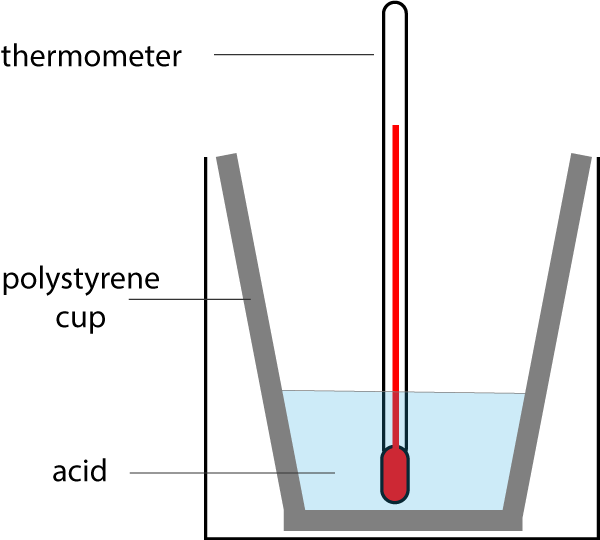1. Which of the following reactions is exothermic?

 Initial temperature / °C Final temperature / °C A 24 19 B 21 23 C 15 3 D 10 -2
2. How do the temperature and energy change in an endothermic reaction?
• A.   temperature decreases and energy is taken in from surroundings
• B.   temperature decreases and energy is given out to surroundings
• C.   temperature increases and energy is taken in from surroundings
• D.   temperature increases and energy is given out to surroundings
3. A student reacted 25cm3 of sodium hydroxide solution with 25cm3 of dilute sulfuric acid. There was no visible change but he observed that the temperature rose by 3°C.

What types of reaction are taking place?

• A.   exothermic and displacement
• B.   exothermic and neutralisation
• C.   endothermic and displacement
• D.   endothermic and neutralisation
4. Which of the following changes of state is exothermic?
• A.   Fe(s)  →  Fe(l)
• B.   H2O(l)  →  H2O(g)
• C.   NH3(g)  →  NH3(l)
• D.   I2(s)  →  I2(g)

Q5-6:
A student investigated the reactivity of four metals W, X, Y and Z by reacting them with copper(II)sulfate solution. The temperature of the solution was recorded before and after reaction:

 Metal Initial temperature / °C Final temperature / °C W 24.3 37.6 X 24.3 24.3 Y 24.3 26.2 Z 24.3 35.9
5. The order of reactivity of the metals , from most to least reactive is:
• A.   W, X, Y, Z
• B.   W, Z, Y, X
• C.   X, Y, Z, W
• D.   Z, Y, X, W
6. Which of the following did the student NOT need to keep the same in each experiment to ensure a fair test?
• A.  the  same volume of copper(II)sulfate solution
• B.   the same concentration of copper(II)sulfate solution
• C.   the same number of moles of each metal
• D.   the same thermometer

7. Which of the following is true about the temperature and the sign of the energy change, for an exothermic reaction?

 Temperature Sign of energy change A decreases negative B decreases positive C increases negative D increases positive

Q8-9:
A student used the following apparatus to measure the temperature change when magnesium ribbon reacted with hydrochloric acid forming magnesium chloride and hydrogen gas.
He noticed that the temperature increased.8. Why did the student use a polystyrene cup rather than a beaker for this experiment?

• A.   polystyrene is cheaper than a beaker
• B.   polystyrene is an insulator
• C.   polystyrene does not break if dropped
• D.   polystyrene does not react with acid

9. Select the energy level diagram which correctly represents this reaction.10. Which of the following is an endothermic reaction?
• A.   combustion of methane gas
• B.   thermal decomposition of calcium carbonate
• C.   oxidation of magnesium
• D.   neutralization of hydrochloric acid with sodium hydroxide